ListCorrelate

The next cell demonstrates the basic use of ListCorrelate.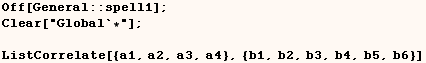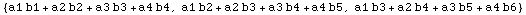Next we see that ListCorrelate is equivalent to flattening the result of a
certain matrix product.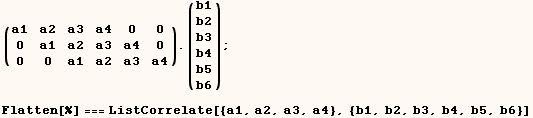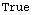The next cell shows that ListConvolve is closely related to ListCorrelate.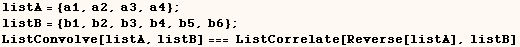Specifying the "overhang" using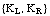In the next cell we provide ListCorrelate {-1,-1} as a third argument.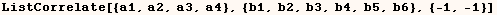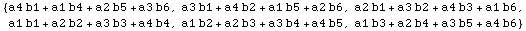The previous example is equivalent to the matrix product in the next cell.
Here we have the last element of {a1, a2, a3, a4} in the upper left position
of the left matrix..  We also have the last element of {a1, a2, a3, a4} in
the lower right position of the left matrix.  The (-1) indicates last element
of {a1, a2, a3, a4}, and (-2) would indicate the second from the last element
of {a1, a2, a3, a4}.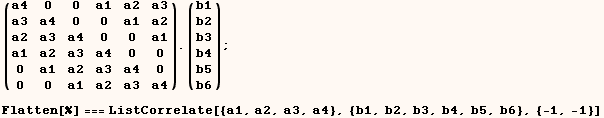In the next cell we provide ListCorrelate {1,1} as a third argument.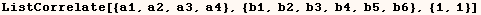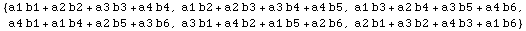The previous example is equivalent to the matrix product in the next cell.
Here we have the first element of {a1, a2, a3, a4} in the upper left position
of the left matrix..  We also have the first element of {a1, a2, a3, a4} in
the lower right position of the left matrix.  The (1) indicates first element
of {a1, a2, a3, a4}, and (2) would indicate the second element of {a1, a2,
a3, a4}.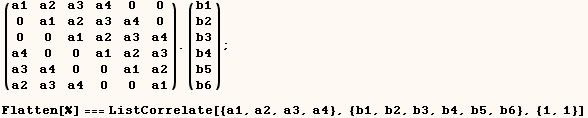In the next cell we provide ListCorrelate {1,-1} as a third argument.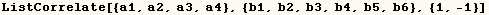The previous example is equivalent to the matrix product in the next cell.
Here we have the first element of {a1, a2, a3, a4} in the upper left position
of the left matrix..  We also have the last element of {a1, a2, a3, a4} in
the lower right position of the left matrix.  The (1) indicates first element
of {a1, a2, a3, a4}, and (-1) indicates the last element of {a1, a2, a3, a4}.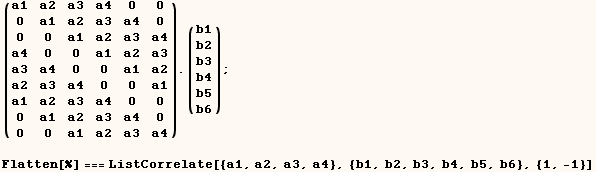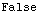In the next cell we provide ListCorrelate {-1,1} as a third argument. Notice
this gives the same result as

ListCorrelate[{a1,a2,a3,a4},{b1,b2,b3,b4,b5,b6}]  (ie. {-1,1} is the default
for the third argument).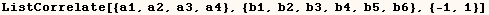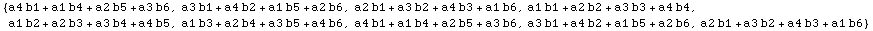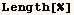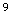The previous example is equivalent to the matrix product in the next cell.
Here we have the last element of {a1, a2, a3, a4} in the upper left position
of the left matrix..  We also have the First element of {a1, a2, a3, a4} in
the lower right position of the left matrix.  The (1) indicates first element
of {a1, a2, a3, a4}, and (-1) indicates the last element of {a1, a2, a3, a4}.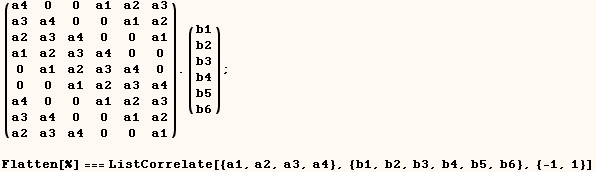In the next cell we provide (s) as a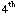argument.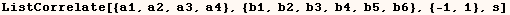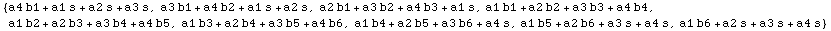The next cell performs a series of Dot products that give the same result as
in the previous cell.  Notice we gave ListCorrelate {1,-1} as a third
argument, and the left part of the first row starts with the first part of
{a1,a2,a3,a4}.  Also the last row ends with the last element of
{a1,a2,a3,a4}.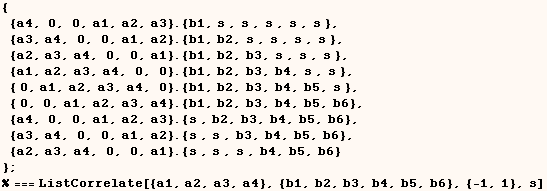In the next cell we provide {s1 s2, s3, s4, s5, s6} as aargument.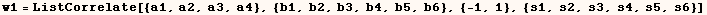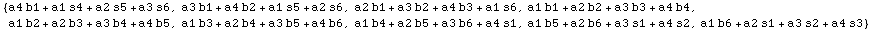The next cell performs a series of Dot products that give the same result  as in the previous cell. Notice the difference between giving (s) and giving  {s,t,u} as aargument.  I actually have a hard time seeing when this would be useful.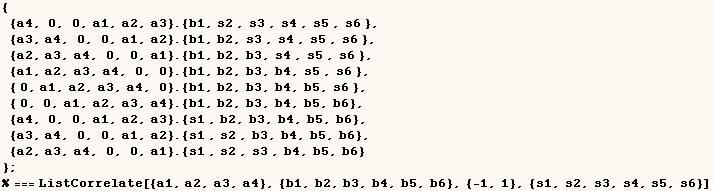To make this more clear I give another example where we give ListCorrelate aargument in the next cell.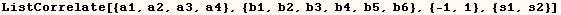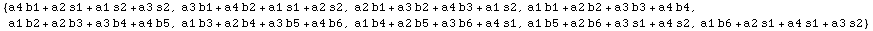The next cell performs a series of Dot products that give the same result as
in the previous cell.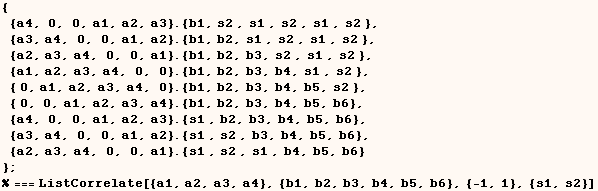It's curious that providing an empty list as aargument is equivalent to providing the integer 1 as aargument.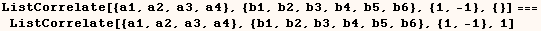The next cell shows that by default ListCorrelate uses the second argument in place of aargument when none is provided.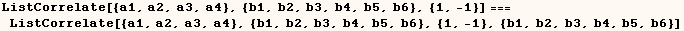Generalizing beyond Times, Plus

In the next cell we provide ListConvolve (g) as a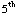argument and it uses the head (g) in place of Times.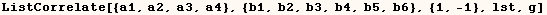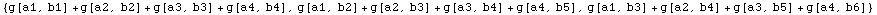In the next cell we provide ListCorrelate (g, h) as aand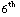arguments respectively and it uses the heads (g) and (h) in place of  Times and Plus respectively.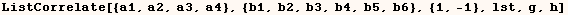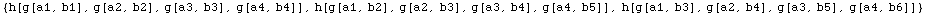ListCorrelate with matrices

In the next cell we perform a ListCorrelate on matrices.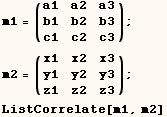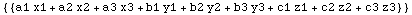The next cell shows how the same ListCorrelate can be done as Dot products on
parts of the matrices.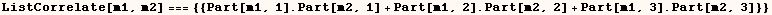We can give ListCorrelate a level specification as a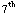argument.  The next cell shows that level (2) is the default  specification when working on matrices.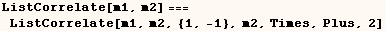In the next cell we give ListCorrelate the integer (1) as a level
specification.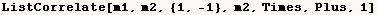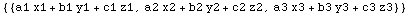The next cell shows how the same ListCorrelate can be done as Dot products on
parts of the matrices.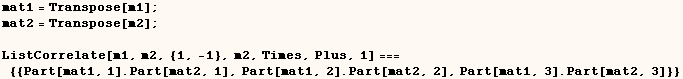The next cell shows two ways of expressing the default for the third argument
of ListCorrelate when working with matrices.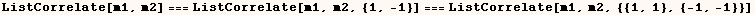I am having a hard time seeing a pattern to how a ListConvolution on matrices
is done in different cases.  I would like to have a complete understanding
for what the third argument of ListCorrelate does when working with matrices,
but it's too hard to decipher.  For example I would like to understand the
result of the examples below.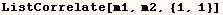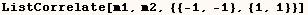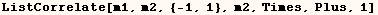I also understand we can also perform a ListCorrelate on tensors, but that is
also too confusing for me to think about.

Created by Mathematica  (May 16, 2004)

Back to Ted’s Tricks index page